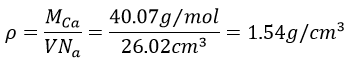Chapter 4c: Solids – crystallography

A characteristic of the solids is that they have their own shape. Liquids are taking the shape of their recipient and gases take all the available space. So solids have their own shape but some are malleable, can be cut, be creased or smashed or can be very rigid. As explained at the beginning of the chapter, all is a question of interactions between the elements of the solid.

There are different types of structure for solids: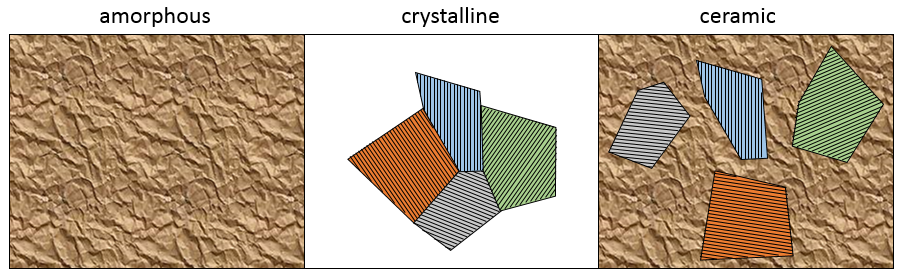Amorphous: disordered arrangement of the atoms

Crystalline: ordered arrangement of atoms. In a monocrystal, the whole solid grew from one point and there is a single arrangement. However, it is difficult to obtain a monocrystal because several growth point can develop simultaneously crystals with different orientations. Several crystals can grow in different directions and coexist in one solid.

Ceramic: some crystals in an amorphous network. It is a melange of the two previous types of structure.

The structure of a solid can be determined through the diffraction of X rays.

The solids can be made of different types of liaisons:

Metallic: electrons can move along the solid. They are very compact and show identical properties in all the directions

Ionic: anions are surrounded by cations and vice versa. In the case of spherical ions, the properties are identical in the three directions.

Covalent: electrons are localised between the nuclei, in the liaisons between atoms. The atoms cannot move freely. It is less dense than ionic or metallic solids because of the imposed liaison length and orientation.

Molecular: there is no specific rule to bind. In molecular solids, the molecules can move easily.

Crystal lattices

The structure of crystals can be defined through the smallest repeatable constituent of the network. NaCl is an ionic solid. Each Na is surrounded by 4 Cl and vice versa. The smallest repeatable constituent of the network is a cube made of 4 Na and 4 Cl, the atoms being in the corners of the cube.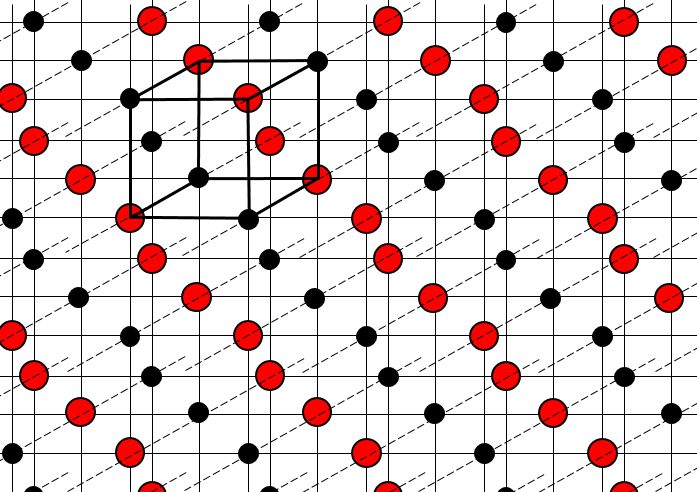There are different base structures for solids. In general, one compound means one crystalline structure. Crystals of salt NaCl have a cubic structure.  Carbon can have two different structures: the graphite (hexagonal) and the diamond (face-centred cubic). In the graphite, there are weak interactions between the sheets of carbon, making them easy to separate.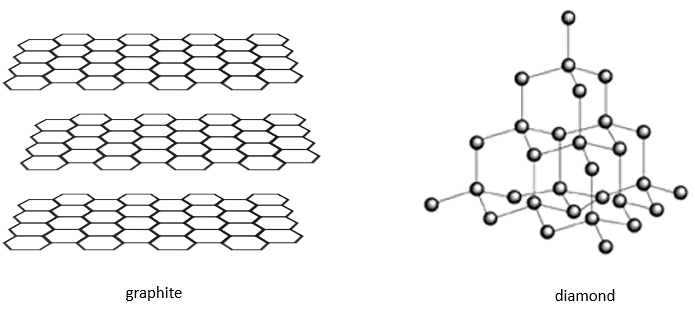While the atoms are the same, the properties of the diamond and of the graphite are very different. Diamonds don’t conduct electricity, don’t break and are translucent. Their colour and opacity depend on impurities. Graphite  conducts electricity (the electron of the orbital p are not bound and can move along the planes), break (planes can slide on each other) and are opaque.

3 parameters are to be considered to sort the crystals: the lengths of the sides (a, b and c) of the structure, the angles between the sides (α, β and γ) and the position of the atoms in the structure. The geometric parameters give 7 lattice systems, given bellow while the position of the atoms sub-sorts them in 14 Bravais lattices

The different types of structures are:

Cubic

In the cubic structure, the lengths of the sides are equal, a=b=c and α=β=γ=90°. If one atom is in the middle of the cube, we call this structure body centred. If one atom is in the middle of each face, we call it face centred.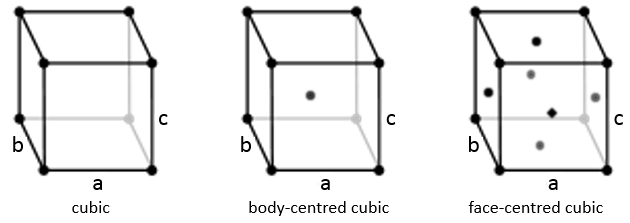Tetragonal

When one side has a different length than the two others (a=b≠c), the structure is called tetragonal. The body-centred also exists for this structure.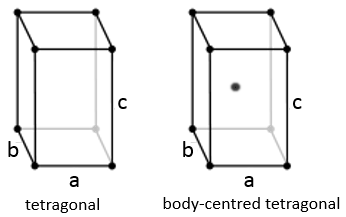Orthorhombic

The angles are still 90° but none of the three characteristic lengths are equal (a≠b≠c). In the case of orthorhombic structures, an additional structure exists: the base-centred structure. In this structure, two opposite sides exhibit an atom at their centre. The other two sides do not.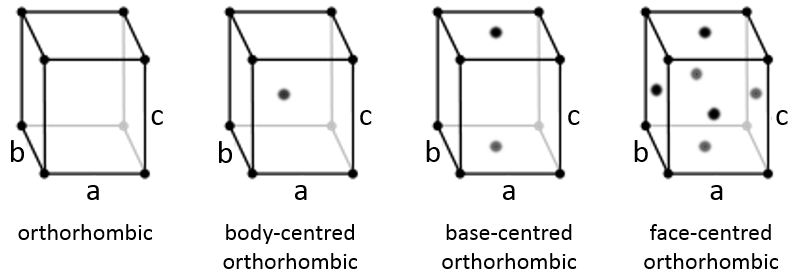Hexagonal

This structure is called hexagonal but none of its face is hexagonal. However, if you assemble three of them you obtain a hexagonal prism. The base and top sides are diamond-shaped (a=b) with an angle γ=120°. The other faces of the structure are rectangular (a=b≠c) or cubic (a=b=c).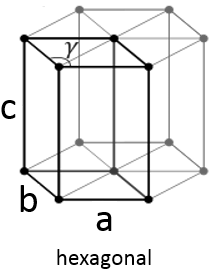Rhombohedral

As for cubic structures, a=b=c and the angles are equal but in a rhombohedral structure they are not right: α=β=γ≠90°.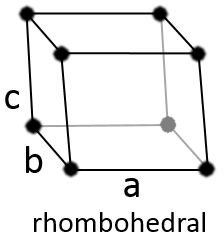Monoclinic

In this case, two angles are equal to 90° but the third angle is not right. The sides have different lengths a≠b≠c.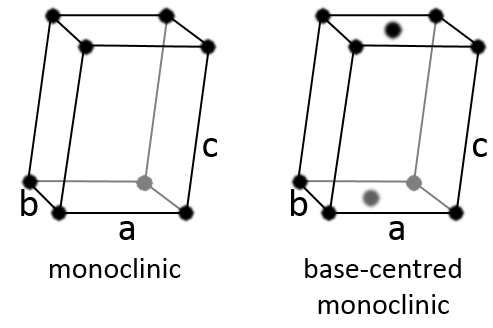Triclinic

The angles α≠β≠γ≠90° are different and there is no right angle. Also, the lengths are different a≠b≠c.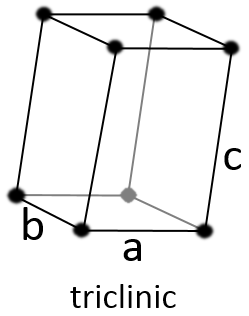Atomic Packing factor

It is determined by the ratio of the volume of the atoms in the crystal and the total volume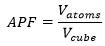Considering spherical atoms, a portion of the cube is inevitably empty. The figures above are schematic and in a real solid, the liaisons are not so long. We would see something like this instead: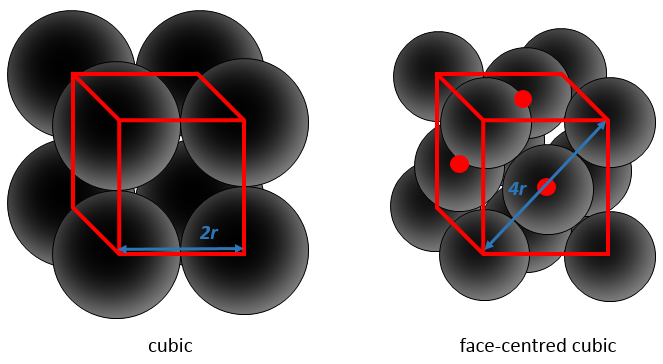This proportion varies from one solid to another one. As an example, let’s consider a face-centred cubic structure wherein all the atoms have the same size, the same radius r. The length of the cube is a and is volume is thus a3.

The length of the diagonal is 4r, and from Pythagoras’ theorem, the square of the hypotenuse equals the sum of the square of the other two sides of a triangle. As a result,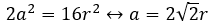We know the total volume of the cube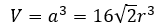We just need to determine how many atoms are in the structure. In each corner of the cube, there is 1/8 of an atom. There are 8 corners, so there is the volume of one atom in total for the corners. At the centre of the faces, there is the half of an atom. There are 6 faces so that makes 3 additional atoms. In total, there are 4 atoms in the cube. The volume of one atom is 4/3 pi r3. The APF for this face-centred cubic is thus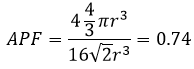For this particular structure, approximately one fourth of the cube is empty. It is the same for the hexagonal structure, reaches one third for the body-centred cubic and one half for the cubic.

• Hexagonal close-packed: 0.74
• Face-centred cubic: 0.74
• Body-centred cubic: 0.68
• Simple cubic: 0.52

Miller indices

Miller indices form a notation system for planes in crystal lattices. It is mainly used in crystallography but I did not used it a lot myself as a chemist. I won’t develop it intensely here. The Miller indices are a trio of 3 integers, written (hkl). Considering one corner of a crystal structure, let’s say a cubic one, as the base (000) of the notation, the axis are following the sides of the crystal. This base is thus not always orthogonal if one of the angles α, β and γ is not right. In the case of a cubic structure, the base is orthogonal.

Moving in the x direction, we will find an atom after a distance a=2r. It is the same if we move in the y or z directions. For the Miller indices, the coordinates x, y and z are divided by the structure dimensions a, b and c respectively.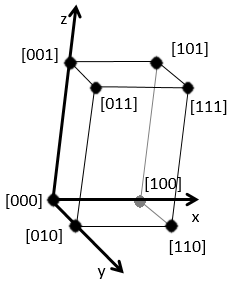The atom on the x axis is on the location  (it is not a Miller index, just a coordinate). The atom on the y axis is on  and the atom on the y axis is on .

The Miller indices (hkl) describe a plane by telling where they cross the axis x, y and z. Considering the following plane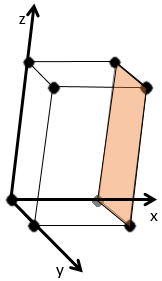We see that it is parallel to the y and z axis and that it crosses the x axis at x=1. As the plane is parallel to y and z, they will never cross. We consider that it would be done at y=∞ and z=∞. To obtain the Miller indices, we take 1 over these values: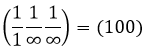If negative values are used, we overstrike them instead of putting a minus sign.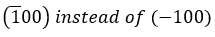Some examples of planes are next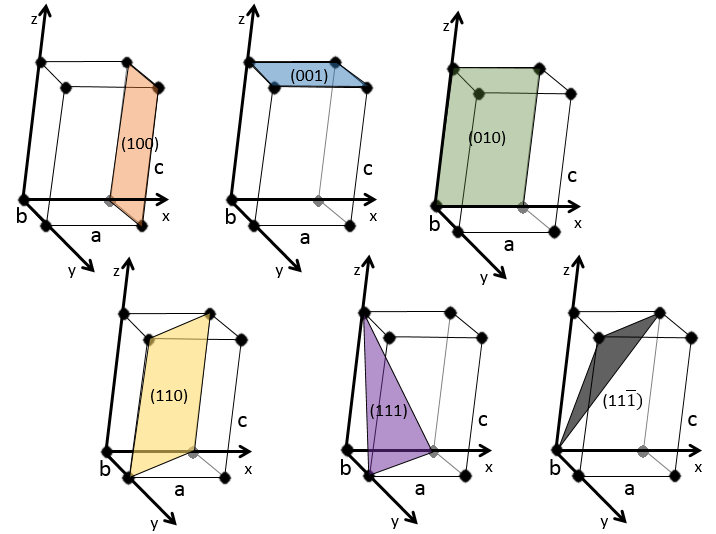Exercises

1.  How do you call these structures?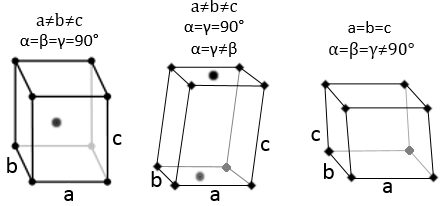2.  Give the Miller indices of the following planes: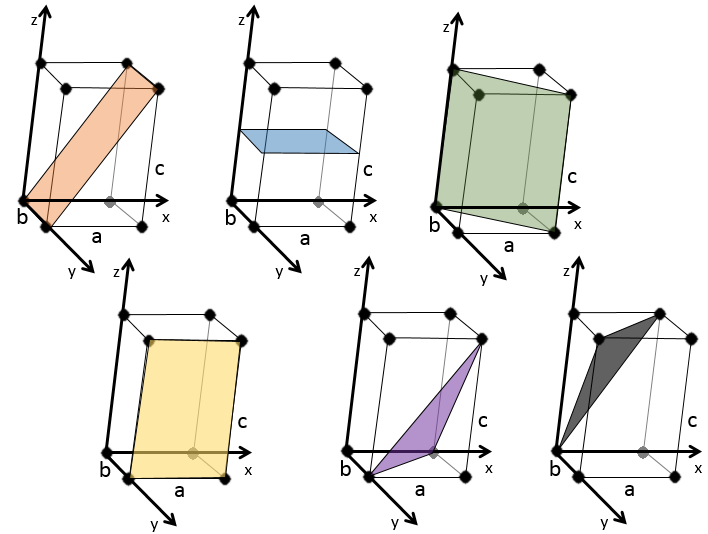3.  The density of the solid NaCl is 2.165. What volume is occupied by 1 mol of it?

4.  Calcium has a fcc configuration. The length of one side of the cube measures 0.557nm. What is the radius of the calcium, the filling ratio and what is the density of this crystal?

1: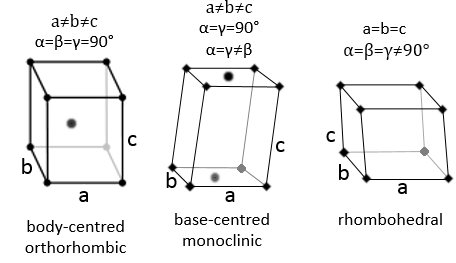2: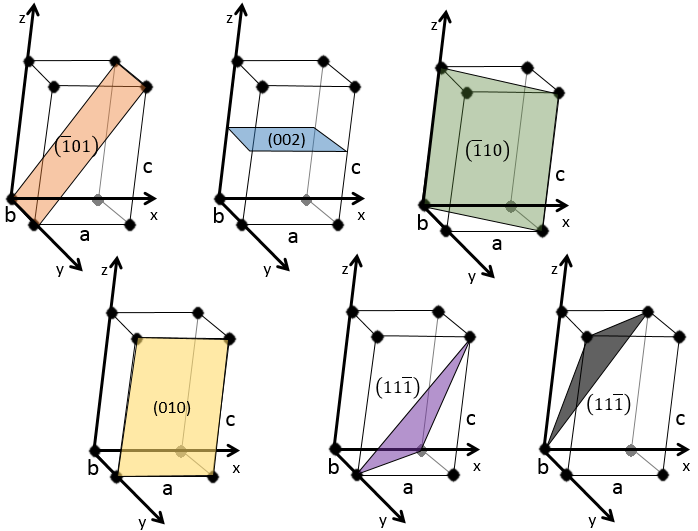For the first one, the showed plane passes by the y axis. Consequently we have to translate it. By default, we translate it following the x axis by one length a to the left.

For the second one, the plane is placed on the middle of the structure, horizontally. Remember that we take the inverse of the position: 1/0.5=2.

As for the first one, the third plane passes by an axis. We translate the plane to obtain the correct answer.

The two last planes have the same Miller indices. They are parallel and merge if we apply a translation in the z direction.

3.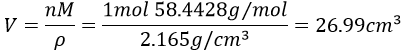4.  The structure is fcc (face-centred cubic) so our point of reference is the diagonal: its length is 4 times the radius of one atom.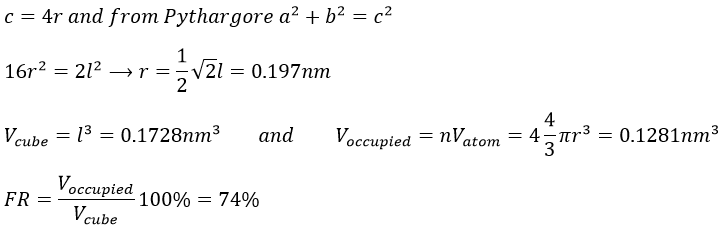To know the density, we calculate the volume of 1 mol